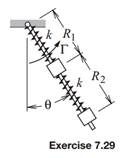### Create an Account

Already have account?

### Forgot Your Password ?

Home / Questions / Use Lagranges equations to solve Exercise 629 Exercise 629 Identical small collars of mas...

# Use Lagranges equations to solve Exercise 629 Exercise 629 Identical small collars of mass m slide with negligible frictional resistance over the bar that is made to rotate in the vertical plane

Use Lagrange’s equations to solve Exercise 6.29.

Exercise 6.29

Identical small collars of mass m slide with negligible frictional resistance over the bar that is made to rotate in the vertical plane by torque Γ that acts about its pivot. The result is that the angle θ is made to vary as a specified function of time. The unstretched length of the identical springs is L. Derive differential equations of motion governing the radial distances R1 and R2.Jun 16 2020 View more View Less

#### Answer (Solved)Subscribe To Get Solution